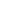# Add a pair of two-digit numbers when the ones digits have a sum greater than 10 using decomposition.

EngageNY 60 min(s)
In this lesson, students add a pair of two-digit numbers when the ones digits have a sum greater than 10 (for example, 49 + 12). In order to solve these problems, they rely on strategies developed in previous units, such as taking apart, making ten, and adding on the tens.